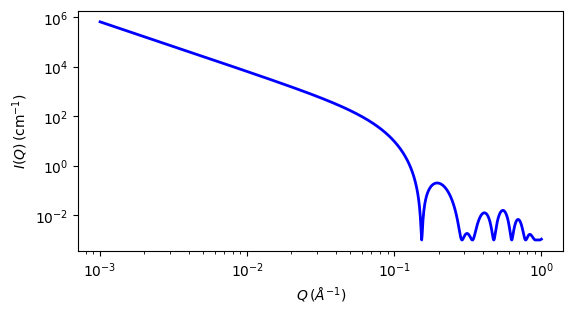# lamellar_hg

Random lamellar phase with Head and Tail Groups

Parameter

Description

Units

Default value

scale

Scale factor or Volume fraction

None

1

background

Source background

cm-1

0.001

length_tail

Tail thickness ( total = H+T+T+H)

15

10

sld

Tail scattering length density

10-6-2

0.4

10-6-2

3

sld_solvent

Solvent scattering length density

10-6-2

6

The returned value is scaled to units of cm-1 sr-1, absolute scale.

This model provides the scattering intensity, $$I(q)$$, for a lyotropic lamellar phase where a random distribution in solution are assumed. The SLD of the head region is taken to be different from the SLD of the tail region.

Definition

The scattering intensity $$I(q)$$ is

$I(q) = 2\pi\frac{\text{scale}}{2(\delta_H + \delta_T)} P(q) \frac{1}{q^2}$

The form factor $$P(q)$$ is

$P(q) = \frac{4}{q^2} \left\lbrace \Delta \rho_H \left[\sin[q(\delta_H + \delta_T)\ - \sin(q\delta_T)\right] + \Delta\rho_T\sin(q\delta_T) \right\rbrace^2$

where $$\delta_T$$ is length_tail, $$\delta_H$$ is length_head, $$\Delta\rho_H$$ is the head contrast (sld_head $$-$$ sld_solvent), and $$\Delta\rho_T$$ is tail contrast (sld $$-$$ sld_solvent).

The total thickness of the lamellar sheet is a_H + delta_T + delta_T + delta_H\$. Note that in a non aqueous solvent the chemical “head” group may be the “Tail region” and vice-versa.

The 2D scattering intensity is calculated in the same way as 1D, where the $$q$$ vector is defined as

$q = \sqrt{q_x^2 + q_y^2}$Fig. 47 1D plot corresponding to the default parameters of the model.

Source

lamellar_hg.py

References

1. F Nallet, R Laversanne, and D Roux, J. Phys. II France, 3, (1993) 487-502

2. J Berghausen, J Zipfel, P Lindner, W Richtering, J. Phys. Chem. B, 105, (2001) 11081-11088

Authorship and Verification

• Author: